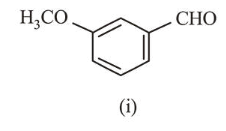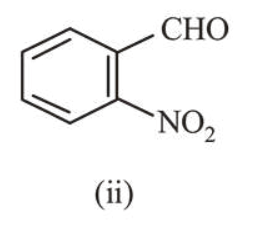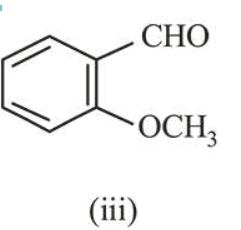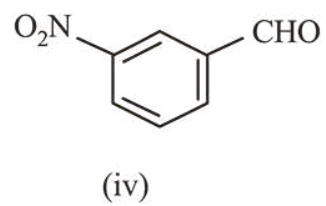# The increasing order of the following compounds towards HCN addition is :

Question:

The increasing order of the following compounds towards HCN addition is :

(1) (i) $<($ iii $)<($ iv $)<($ ii $) (2) (iii)$<($iv$)<($i$)<($ii$)$(3) (iii)$<($i$)<$(iv)$<$(ii) (4) (iii)$<($iv$)<($ii$)<($i$)$1.2.3.4.Correct Option: , 3 Solution: -I effect of$\mathrm{NO}_{2}$increases reactivity towards nucleophilic addition reaction with$\mathrm{HCN} .-\mathrm{OCH}_{3}\$ group is electron donating due to resonance effect which decreases the reactivity towards nucleophillic addition.